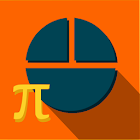Math Fundamentals

• SEARCH TYPE

All Android applications categories

All Android games categories# Math Fundamentals

0 0

## Screenshots

Description

Math Fundamentals has lessons(tutorial with solved examples),practice & test sections on various topics like Number & Operation, Fraction,Decimal,Percentage,Measurement,Number Systems,LCM, Ratio, Time,Money, Interest, Probability,Statistics, Exponent,Series, Basic Algebra , Basic Geometry.

Practice & Test section has hundreds of questions now. But we are adding more. While you practice, you can get the correct answer of each question with explanation. After you do practice, try the test section. In test you need to solve 10 questions in 15min time. Only to know the solution is not enough, you need to do it fast too, Is not it? So our test section will prepare you to solve the question fast.

We have included different types of answer options like fill in blanks,true false,multiple choice,picker,single selection.

Tags: zoom math 200 lcm